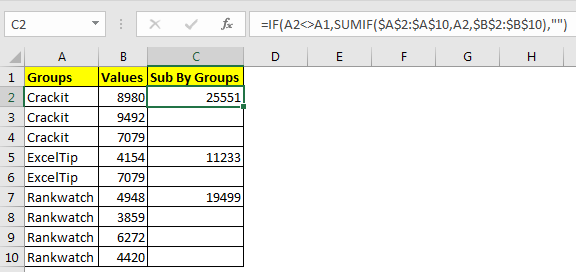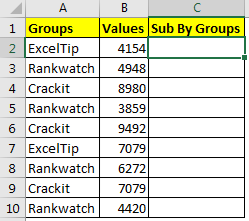# Sum by Groups in The Excel TableIts easy to sum by group outside the table using SUMIF function, but what if we want to sum the groups in the table. Same as pivot table. This also easy to do. This article tells about how to sum values by their group in the same table without repetition.

Generic Formula

=IF(group_name<>Cell_Above_group_name,SUMIF(group_range,group_name,sum_range),””)

The generic formula above may look lengthy but I have just used descriptive names so that it explains it self.
Group_name: the cell reference of name of the group that you want to sum.
Cell_Above_group_name: the cell reference of cell above the group name.
Group_range: the reference of range that contains the group names.
Sum_range: the range of cell that contains the values.

So let’s see how it works with an example.

Example: Sum Values By Group
Here we have this excel table that contains data of three companies with some random data.First and best method is to use pivot tables. Pivot table is best to get summarized and structured data by groups.
But for any reason if you want to sum values by group in the original table only. Then use this method. First sort the table by groups. And then write this formula in C2:

=IF(A2<>A1,SUMIF(\$A\$2:\$A\$10,A2,\$B\$2:\$B\$10),"")

Copy down this formula and we have our sum of different groups.So how this excel formula works?
For this formula to work, sorting of data by groups is very important.

Let’s look at function in C2 =IF(A2<>A1,SUMIF(\$A\$2:\$A\$10,A2,\$B\$2:\$B\$10),"").,
Here the IF function checks if Name in A2 is not equal to A1. They are not equal, hence SUMIF function runs and sums value in range \$B\$2:\$B\$10 for value in A2 only which gives us 25551 in C2.

Let’s look at the function in C3, =IF(A2<>A1,SUMIF(\$A\$2:\$A\$10,A3,\$B\$2:\$B\$10),"")
Here IF function checks if Name in A3 is not equal to A2. They are equal. Hence SUMIF function is skipped and False part is returned which contains nothing (“”).

Related Article:

How to Use SUMIF Function in Excel

SUMIFS with dates in Excel

SUMIF with non-blank cells

How to Use SUMIFS Function in Excel

SUMIFS using AND-OR logic

Popular Articles:

The VLOOKUP Function in Excel

COUNTIF in Excel 2016

How to Use SUMIF Function in Excel

Terms and Conditions of use

The applications/code on this site are distributed as is and without warranties or liability. In no event shall the owner of the copyrights, or the authors of the applications/code be liable for any loss of profit, any problems or any damage resulting from the use or evaluation of the applications/code.